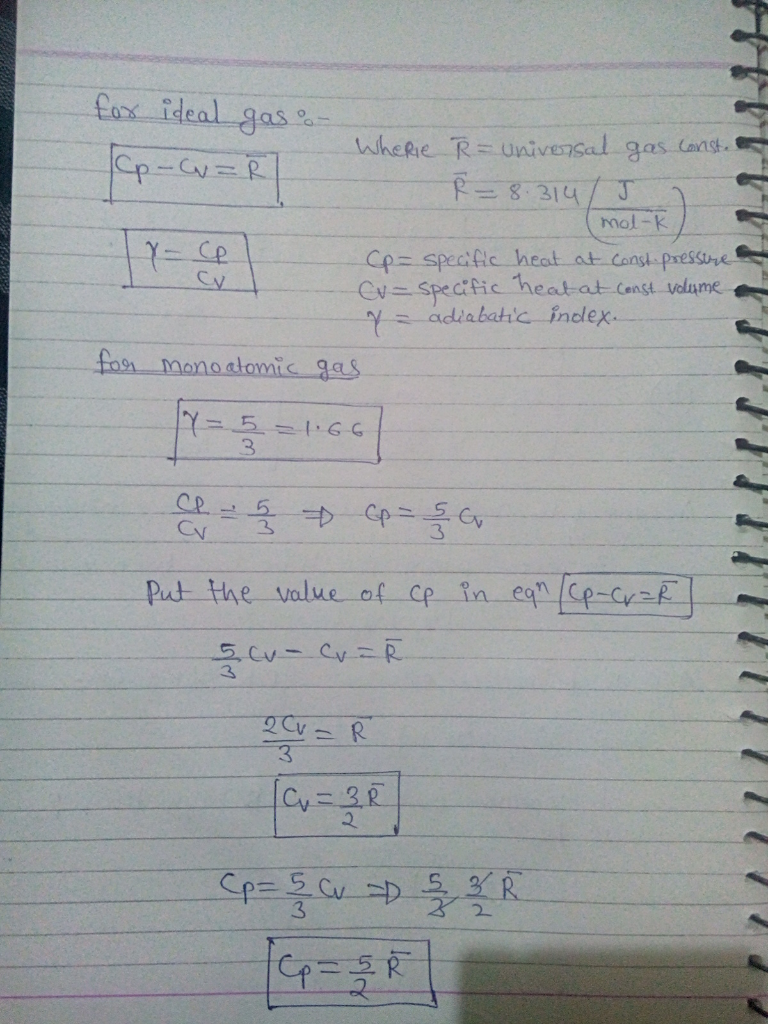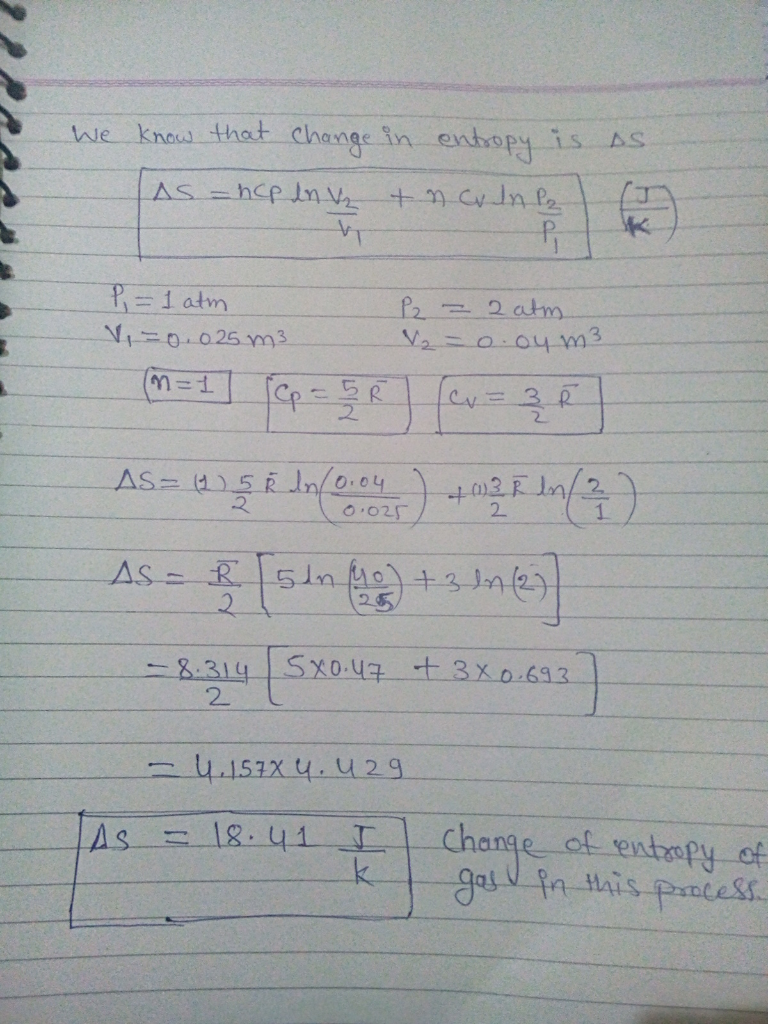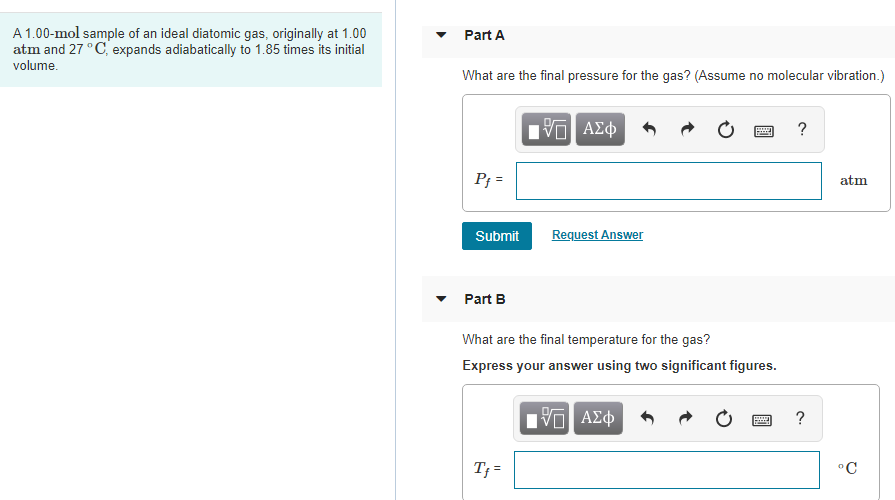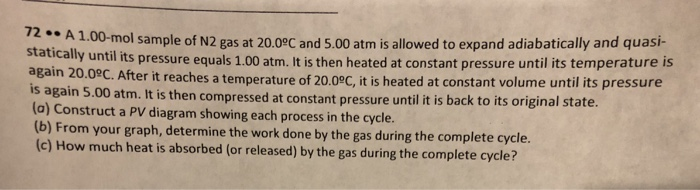Question

# A 1.00-mol sample of an ideal monatomic gas, initially at a pressure of 1.00 atm and...

A 1.00-mol sample of an ideal monatomic gas, initially at a pressure of 1.00 atm and a volume of 0.025 0 m3 , is heated to a final state with a pressure of 2.00 atm. and a volume of 0.040 0 m3 . Determine the change in entropy of the gas in this process.#### Earn Coins

Coins can be redeemed for fabulous gifts.

Similar Homework Help Questions
• ### One mole of an ideal monatomic gas, initially at a pressure of 1.04 atm and a...

One mole of an ideal monatomic gas, initially at a pressure of 1.04 atm and a volume of 0.0124 m^3 , , is heated to a final state where the pressure is 3.04 atm and the volume is 0.0274 m^3 . The gas constant is 8.31447 J/mol · K . Determine the change in entropy for this

• ### Expand 1.00 mol of a monatomic gas, initially at 7.00 kPa and 737 K, from initial...

Expand 1.00 mol of a monatomic gas, initially at 7.00 kPa and 737 K, from initial volume Vi = 0.875 m3 to final volume Vf = 2.90 m3. At any instant during the expansion, the pressure p and volume V of the gas are related by p = 7.00 exp[(Vi - V)/a], with p in kilopascals, Vi and V are in cubic meters, and a = 2.40 m3. What are the final (a) pressure and (b) temperature of the gas?...

• ### Expand 1.00 mol of a monatomic gas, initially at 7.00 kPa and 737 K, from initial...

Expand 1.00 mol of a monatomic gas, initially at 7.00 kPa and 737 K, from initial volume Vi = 0.875 m3 to final volume Vf = 2.90 m3. At any instant during the expansion, the pressure p and volume V of the gas are related by p = 7.00 exp[(Vi - V)/a], with p in kilopascals, Vi and V are in cubic meters, and a = 2.40 m3. What are the final (a) pressure and (b) temperature of the gas?...

• ### A 1.00 mole sample of an ideal monatomic gas, originally a pressure of 1.00 atm, undergoing...

A 1.00 mole sample of an ideal monatomic gas, originally a pressure of 1.00 atm, undergoing a three-step process: • Expands adiabatically from T1 = 588 K to T2 = 300 K • It is compressed at constant pressure until its temperature reaches T3; • Then it returns to its original pressure and temperature using a constant volume process. Calculate cycle efficiency Select one: (Quickly, please :() Calculate cycle efficiency Select one: to. 30.4% b. None of the above options...

• ### 2.00 mol of neon is expanded isothermically at room temperature from 2.00 atm to 1.00 atm...

2.00 mol of neon is expanded isothermically at room temperature from 2.00 atm to 1.00 atm and is then heated from room temperature to 125 C at a constant pressure of 1.00 atm. a) What is the change in entropy for the overall process? b) What is the change in entropy for the process with the same initial and final states as in part (a), but in which the gas is first heated at constant pressure and then expanded isothermically?...

• ### Part A A 1.00-mol sample of an ideal diatomic gas, originally at 1.00 atm and 27°C,...Part A A 1.00-mol sample of an ideal diatomic gas, originally at 1.00 atm and 27°C, expands adiabatically to 1.85 times its initial volume. What are the final pressure for the gas? (Assume no molecular vibration.) 0 AM O O ? Pf= atm Submit Request Answer Part B What are the final temperature for the gas? Express your answer using two significant figures. 10 ADC 0 2 ? T;

• ### A 2.60-mol sample of helium gas initially at 300 K, and 0.400 atm is compressed isothermally...

A 2.60-mol sample of helium gas initially at 300 K, and 0.400 atm is compressed isothermally to 1.00 atm. Note that the helium behaves as an ideal gas. (a) Find the final volume of the gas.? m3 (b) Find the work done on the gas. kJ (c) Find the energy transferred by heat. kJ

• ### An ideal monatomic gas initially has a temperature of 267 K and a pressure of 6.14...

An ideal monatomic gas initially has a temperature of 267 K and a pressure of 6.14 atm. It is to expand from volume 488 cm3 to volume 1610 cm3. If the expansion is isothermal, what are (a) the final pressure and (b) the work done by the gas? If, instead, the expansion is adiabatic, what are (c) the final pressure and (d) the work done by the gas?

• ### A container holds 4.5 mol of an ideal monatomic gas with a pressure of 125 kPa....

A container holds 4.5 mol of an ideal monatomic gas with a pressure of 125 kPa. The container initially has a volume of 0.10 m3. The gas undergoes an adiabatic expansion until it reaches a volume of 0.3 m3 and a pressure of 20.0 kPa. What is the thermal energy of the gas after the expansion? How much energy went into or out of the gas as work during the expansion? (Positive for energy into the gas, negative for energy...

• ### 1.00-mol sample of N2 gas at 20.09C and 5.00 atm is allowed to expand adiabatically and...1.00-mol sample of N2 gas at 20.09C and 5.00 atm is allowed to expand adiabatically and quasi- C. After it reaches a temperature of 20.09C, it is heated at constant volume until its pressure 72..A stati cally until its pressure equals 1.00 atm. It is then heated at constant pressure until its temperature is is again 5.00 (a) Construct a PV diagram showing each process in the cycle. (b) From your graph, determine the work done by the gas during...